# dsp.ArrayPlot

Display vectors or arrays

## Description

Display vectors or arrays where the data is uniformly spaced along the x-axis.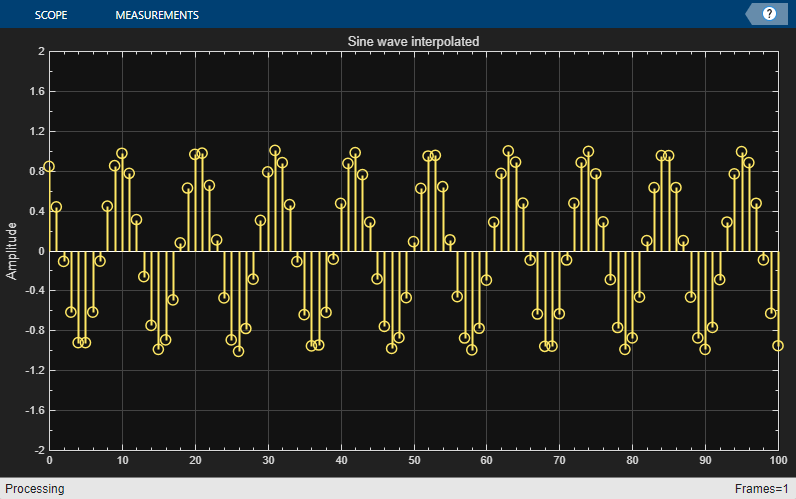## Creation

### Syntax

``scope = dsp.ArrayPlot``
``scope = dsp.ArrayPlot(Name,Value)``

### Description

example

````scope = dsp.ArrayPlot` creates an Array Plot object, `scope`.`scope = dsp.ArrayPlot(Name,Value)` sets properties using one or more name-value pairs. Enclose each property name in quotes. For example, ```scope = dsp.ArrayPlot("NumInputPorts",3)``````

## Properties

expand all

Most properties can be changed from the `dsp.ArrayPlot` UI.

### Plot Configuration

Number of input ports, specified as a positive integer. Each signal coming through a separate input becomes a separate channel in the scope. You must invoke the scope with the same number of inputs as the value of this property.

Specify whether to use the `SampleIncrement` and `XOffset` property values to determine spacing, or specify your own custom spacing. If you specify `"Custom"`, you also must specify the `CustomXData` property values.

You can set this property only when creating the object.

#### UI Use

Open the Plot tab, click Settings, and set X-Data Mode.

Data Types: `char` | `string`

Specify the desired x-data values as a row or column vector of length equal to the frame length of the inputs. If you use the default (empty vector) value, the x-data is uniformly spaced and set to (0:L–1), where L is the frame length.

You can set this property only when creating the object.

Example: ```scope = dsp.ArrayPlot("XDataMode","Custom","CustomXData",logspace(0,log10(44100/2),1024))```

#### UI Use

Open the Plot tab, click Settings, and set X-Data Mode to Custom and specify Custom X-Data.

#### Dependency

To use this property, set `XDataMode` to `"Custom"`.

Specify the spacing between samples along the x-axis as a finite numeric scalar. The input signal is only y-axis data. x-axis data is set automatically based on the `XOffset` and `SampleIncrement` properties.

Example: When `XOffset` is `0` and `SampleIncrement` is `1`, the x-axis values are set to `0, 1, 2, 3, 4, …` .

Example: When `XOffset` is `-1` and `SampleIncrement` is `0.25`, the x-axis values are set to ```-1, -0.75, -0.5, -0.25, 0, …``` .

#### UI Use

Open the Plot tab, click Settings, and set Sample Increment.

#### Dependency

To use this property, set `XDataMode` to ```'Sample increment and X-offset'```.

Display offset of x-axis, specified as a numeric scalar. x-axis data is set automatically based on both the `SampleIncrement` and `XOffset` values. The x-offset represents the first value on the x-axis.

Example: When `XOffset` is 0 and `SampleIncrement` is 1, the x-axis values are set to 0, 1, 2, 3, 4, … .

Example: When `XOffset` is -1 and `SampleIncrement` is 0.25, the x-axis values are set to -1, -0.75, -0.5, -0.25, 0, … .

#### UI Use

Open the Plot tab, click Settings, and set X-Offset.

#### Dependency

To use this property, set `XDataMode` to ```'Sample increment and X-offset'```.

Specify whether the scale of the x-axis is `"Linear"` or `"Log"`. If `XOffset` is a negative value, you cannot set this property to `"Log"`.

#### UI Use

Open the Plot tab, click Settings, and set XScale.

Data Types: `char` | `string`

Specify whether the scale of the y-axis is `"Linear"` or `"Log"`.

#### UI Use

Open the Plot tab, click Settings, and set YScale.

Data Types: `char` | `string`

Specify the type of plot to use for all the input signals displayed in the scope window:

• `"Stem"` – The scope displays the input signal as circles with vertical lines extending down to the x-axis at each of the sampled values.

• `"Line"` – The scope displays the input signal as lines connecting each of the sampled values.

• `"Stairs"` – The scope displays the input signal as a stair-step graph. A stair-step graph is made up of only horizontal lines and vertical lines. Each horizontal line represents the signal value for a discrete sample period and is connected to two vertical lines. Each vertical line represents a change in values occurring at a sample. Stair-step graphs are useful for drawing time history graphs of digitally sampled data.

#### UI Use

Open the Settings and set Plot Type.

Specify when the scope scales the axes. Valid values are:

• `"Auto"` — The scope scales the axes as needed to fit the data, both during and after simulation.

• `"Manual"` — The scope does not scale the axes automatically.

• `"OnceAtStop"` — The scope scales the axes when the simulation stops.

• `"Updates"` — The scope scales the axes once and only once after 10 updates.

You can set this property only when creating the object.

#### UI Use

Hover over the array plot to see the zoom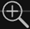, pan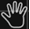, and autoscale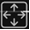buttons. You can also zoom and pan using your mouse.

Data Types: `char` | `string`

### Visualization

Specify the name of the scope. This name appears as the title of the scope's figure window. To specify a title of a scope plot, use the `Title` property.

Data Types: `char` | `string`

Specify, in pixels, the size and location of the scope window as a four-element vector of the form ```[left bottom width height]```. By default, the scope window appears in the center of your screen with a width of 800 pixels and height of 450 pixels. The default values for this property may change depending on your screen resolution.

Specify whether to display the scope in maximized-axes mode. In this mode, the axes are expanded to fit into the entire display. To conserve space, labels do not appear in each display. Instead, tick-mark values appear on top of the plotted data. You can select one of the following options:

• `"Auto"` — The axes appear maximized in all displays only if the `Title` and `YLabel` properties are empty for every display. If you enter any value in any display for either of these properties, the axes are not maximized.

• `"On"` — The axes appear maximized in all displays. Any values entered into the `Title` and `YLabel` properties are hidden.

• `"Off"` — None of the axes appear maximized.

#### UI Use

Hover over the array plot to see the maximize axes button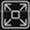.

Data Types: `char` | `string`

Specify the display title as a character vector or string.

#### UI Use

Open the Plot tab, click Settings, and set Title.

Data Types: `char` | `string`

To show a legend with the input names, set this property to `true`.

From the legend, you can control which signals are visible. This control is equivalent to changing the visibility in the Style dialog box. In the scope legend, click a signal name to hide the signal in the scope. To show the signal, click the signal name again. To show only one signal, right-click the signal name. To show all signals, press Esc.

Note

The legend only shows the first 20 signals. Any additional signals cannot be viewed or controlled from the legend.

#### UI Use

On the Plot tab, click .

Data Types: `logical`

Specify the input channel names as a cell array of character vectors. The names appear in the legend, Settings, and Measurements panels. If you do not specify names, the channels are labeled as `Channel 1`, `Channel 2`, etc.

#### Dependency

To see channel names, set `ShowLegend` to `true`.

Data Types: `char`

Set this property to `true` to show grid lines on the plot.

#### UI Use

Open the Plot tab, click Settings, and select Grid.

• `true` – The scope plots the magnitude and phase of the input signal on two separate axes within the same active display.

• `false` – The scope plots the real and imaginary parts of the input signal on two separate axes within the same active display.

This property is useful for complex-valued input signals. Turning on this property affects the phase for real-valued input signals. When the amplitude of the input signal is nonnegative, the phase is 0 degrees. When the amplitude of the input signal is negative, the phase is 180 degrees.

#### UI Use

On the Plot tab, select the Magnitude Phase button.

Specify the text for the scope to display below the x-axis.

#### UI Use

Open the Plot tab, click Settings, and set XLabel.

Data Types: `char` | `string`

Specify the text for the scope to display to the left of the y-axis.

#### Dependencies

This property applies only when `PlotAsMagnitudePhase` is `false`. When `PlotAsMagnitudePhase` is `true`, the two y-axis labels are read-only values `"Magnitude"` and `"Phase"`, for the magnitude plot and the phase plot, respectively.

#### UI Use

Open the Plot tab, click Settings, and set YLabel.

Data Types: `char` | `string`

Specify the y-axis limits as a two-element numeric vector, `[ymin, ymax]`.

If `PlotAsMagnitudePhase` is `false`, the default is `[-10,10]`. If `PlotAsMagnitudePhase` is `true`, the default is `[0,10]`.

#### Dependencies

When `PlotAsMagnitudePhase` is `true`, this property specifies the y-axis limits of only the magnitude plot. The y-axis limits of the phase plot are always `[-180,180]`.

#### UI Use

Open the Plot tab, click Settings, and set Y-Axis Limits as a two-element numeric vector.

## Usage

### Syntax

``scope(signal)``
``scope(signal1,signal2,...,signalN)``

### Description

example

````scope(signal)` displays the signal in the Array Plot.`scope(signal1,signal2,...,signalN)` displays multiple signals in the Array Plot. The signals must have the same frame length, but can vary in number of channels. You must set the `NumInputPorts` property to enable multiple input signals. ```

### Input Arguments

expand all

Specify one or more input signals to visualize in the `dsp.ArrayPlot`. Signals can have a different number of channels, but they must have the same frame length.

Example: `scope(signal1, signal2)`

#### UI Customization

To customize the style of signals on the array plot, open the and use the bottom row of options to select a signal and modify the style, width, color, and marker type.

Data Types: `single` | `double` | `int8` | `int16` | `int32` | `int64` | `uint8` | `uint16` | `uint32` | `uint64` | `fi`

## Object Functions

To use an object function, specify the object as the first input argument.

 `generateScript` Generate MATLAB script to create scope with current settings `hide` Hide scope window `show` Display scope window `isVisible` Determine visibility of scope `step` Run System object algorithm `release` Release resources and allow changes to System object property values and input characteristics `reset` Reset internal states of System object

## Examples

collapse all

Note: This example runs only in R2016b or later. If you are using an earlier release, replace each call to the object with the equivalent `step` syntax. For example, `myObject(x)` becomes `step(myObject,x)`.

Create a new Array Plot object.

```scope = dsp.ArrayPlot; ```

Configure the properties of the Array Plot object for a Gaussian distribution.

```scope.YLimits = [0 1]; scope.XOffset = -2.5; scope.SampleIncrement = 0.1; scope.Title = 'Gaussian distribution'; scope.XLabel = 'X'; scope.YLabel = 'f(X)'; ```

Call the Array Plot object to plot a Gaussian distribution.

```scope(exp(-(-2.5:.1:2.5).*(-2.5:.1:2.5))') ```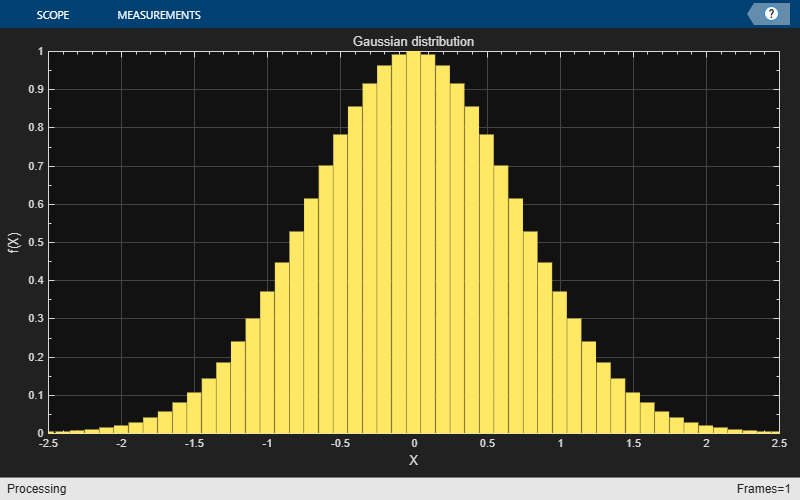Note: This example runs only in R2016b or later. If you are using an earlier release, replace each call to the object with the equivalent `step` syntax. For example, `myObject(x)` becomes `step(myObject,x)`.

View least mean squares (LMS) adaptive filter weights on the Array Plot figure. Watch the filter weights change as they adapt to filter a noisy input signal.

Create an LMS adaptive filter System object.

```lmsFilter = dsp.LMSFilter(40,'Method','Normalized LMS','StepSize',0.002); ```

Create and configure a dsp.AudiFileReader System object to read the input signal from the specified audio file.

```signalSource = dsp.AudioFileReader('dspafxf_8000.wav',... 'SamplesPerFrame',40, ... 'PlayCount',Inf,... 'OutputDataType','double'); ```

Create and configure a dsp.FIRFilter System object to filter random white noise, creating colored noise.

```firFilter = dsp.FIRFilter('Numerator',fir1(39,0.25)); ```

Create and configure an Array Plot System object to display the adaptive filter weights.

```scope = dsp.ArrayPlot('XLabel','Filter Tap', ... 'YLabel','Filter Weight', ... 'YLimits',[-0.05 0.2]'); ```

Plot the LMS filter weights as they adapt to a desired signal. Read from the audio file, produce random data, and filter the random data. Update the filter weights and plot the filter weights.

```numplays = 0; while numplays < 3 [y, eof] = signalSource(); noise = rand(40,1); noisefilt = firFilter(noise); desired = y + noisefilt; [~, ~, wts] = lmsFilter(noise,desired); scope(wts); numplays = numplays + eof; end ```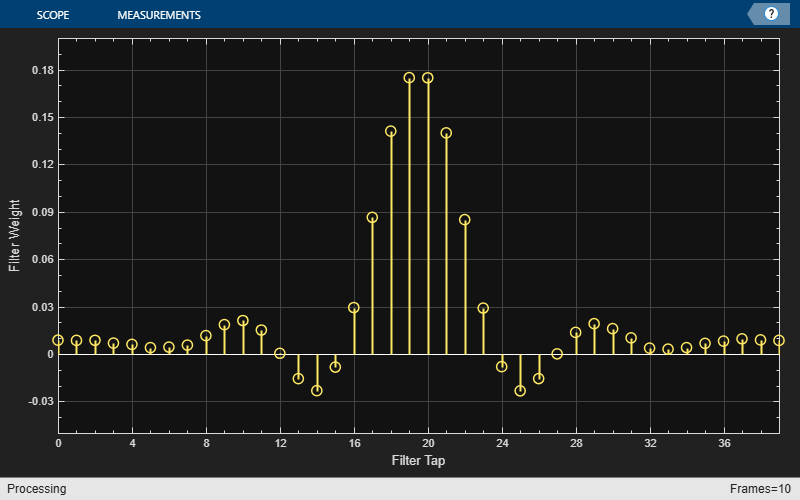## Extended Capabilities

### Topics

Introduced in R2013a

## SupportGet trial now